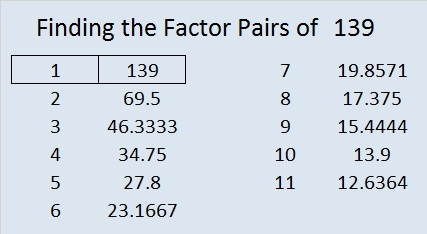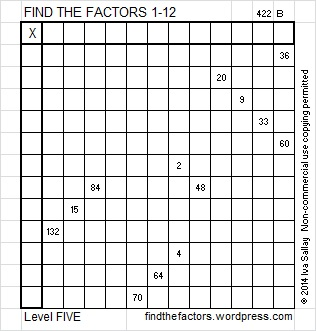# 139 and Level 5

• 139 is a prime number.
• Prime factorization: 139 is prime.
• The exponent of prime number 139 is 1. Adding 1 to that exponent we get (1 + 1) = 2. Therefore 139 has exactly 2 factors.
• Factors of 139: 1, 139
• Factor pairs: 139 = 1 x 139
• 139 has no square factors that allow its square root to be simplified. √139 ≈ 11.789826How do we know that 139 is a prime number? If 139 were not a prime number, then it would be divisible by at least one prime number less than or equal to √139 ≈ 11.8. Since 139 cannot be divided evenly by 2, 3, 5, 7, or 11, we know that 139 is a prime number.

139 is never a clue in the FIND THE FACTORS puzzles.Excel file with puzzles and the previous week’s factor solutions: 12 Factors 2014-06-02This site uses Akismet to reduce spam. Learn how your comment data is processed.